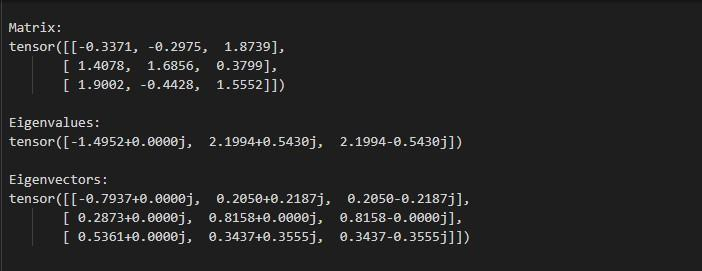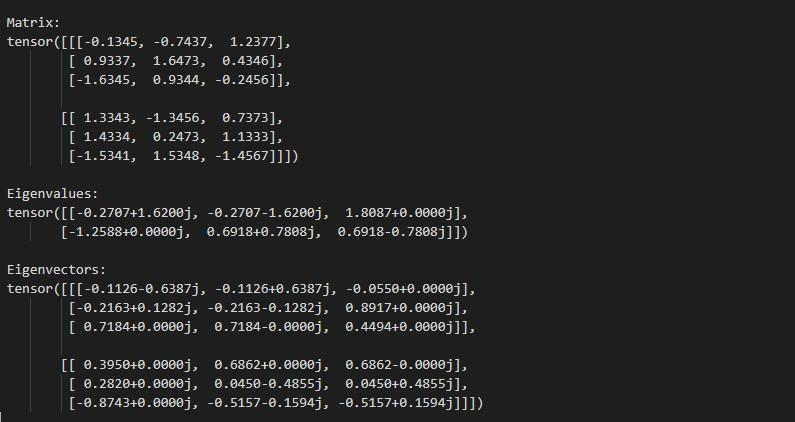GeeksforGeeks App
Open AppBrowser
Continue

# How to compute the eigenvalues and eigenvectors of a square matrix in PyTorch?

In this article, we are going to discuss how to compute the eigenvalues and eigenvectors of a square matrix in PyTorch.

## Compute the eigenvalues and eigenvectors of a square matrix in PyTorch

torch.linalg.eig() method computes the eigenvalue decomposition of a square matrix or a batch of matrices. The decomposition exists if the input matrix is diagonalizable. This method also supports the input of float, double, cfloat, and cdouble data types. It will return a named tuple (eigenvalues, eigenvectors). The eigenvalues and eigenvectors are always complex-valued and the eigenvectors are given by columns of eigenvectors. Below is the syntax of torch.linalg.eig() method.

Syntax: torch.linalg.eig(mat)

Parameter:

• mat (Tensor): square matrix or a batch of matrices.

Return: It will return a named tuple (eigenvalues, eigenvectors).

Example 1:

In this example, we see how to compute the eigenvalues and eigenvectors of a square matrix.

## Python3

 `# import the required library``import` `torch`` ` `# define a 3x3 square matrix``mat ``=` `torch.tensor([[``-``0.3371``, ``-``0.2975``, ``1.8739``],``                    ``[``1.4078``, ``1.6856``, ``0.3799``],``                    ``[``1.9002``, ``-``0.4428``, ``1.5552``]])`` ` `# print the above created matrix``print``(``"\n Matrix: \n"``, mat)`` ` `# compute the eigenvalues and eigenvectors``eigenvalues, eigenvectors ``=` `torch.linalg.eig(mat)`` ` `# print output``print``(``"\n Eigenvalues: \n"``, eigenvalues)``print``(``"\n Eigenvectors: \n"``, eigenvectors)`

Output:EigenValues and EigenVectors

Example 2:

In this example, we see how to compute the eigenvalues and eigenvectors of a batch of matrices.

## Python3

 `# import the required library``import` `torch`` ` `# define a batch of matrices``mat ``=` `torch.tensor([[[``-``0.1345``, ``-``0.7437``, ``1.2377``],``                     ``[``0.9337``, ``1.6473``, ``0.4346``],``                     ``[``-``1.6345``, ``0.9344``, ``-``0.2456``]],``                    ``[[``1.3343``, ``-``1.3456``, ``0.7373``],``                     ``[``1.4334``, ``0.2473``, ``1.1333``],``                     ``[``-``1.5341``, ``1.5348``, ``-``1.4567``]]])`` ` `# print the above batch of matrices``print``(``"\n Matrix: \n"``, mat)`` ` `# compute the eigenvalues and eigenvectors``eigenvalues, eigenvectors ``=` `torch.linalg.eig(mat)`` ` `# print output``print``(``"\n Eigenvalues: \n"``, eigenvalues)``print``(``"\n Eigenvectors: \n"``, eigenvectors)`

Output:EigenValues and EigenVectors

My Personal Notes arrow_drop_up